# AP Chemistry : Gas Laws

## Example Questions

← Previous 1

### Example Question #1 : Gas Laws

What is the final pressure of a gas initially has a pressure of 10 atm at 50 L if the volume s now 25 L?

5 atm

20 atm

50 atm

10 atm

25 atm

20 atm

Explanation:

Use P1V1 = P2V2

P1 = 10atm; V1 = 50L

P2 = X; V2 = 25L

(10atm)(50L) = (x)(25L)

500 = 25x

x = 20

### Example Question #1 : Gas Laws

A sample of ideal gas is heated in a 2L vessel at a temperature of 320 Kelvin. The pressure in the vessel is 2.5atm. What is the new pressure in the vessel if the volume is halved and the temperature is reduced to 250 Kelvin?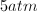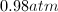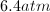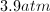Explanation:

Since the gas is ideal, we can use a variation of the ideal gas law in order to find the unknown final pressure.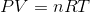Since we know that the number of moles is constant between both vessels (and R is a constant as well), we can simply compare the three factors being manipulated between the two vessels: pressure, volume, and temperature. Using a combination of Boyle's law and Charles's law, we can compare the two vessels to one another using the following equation.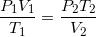Use the given values to solve for the final pressure.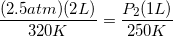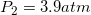### Example Question #12 : Gases

An ideal gas takes up 60L at 2 atm. If the gas is compressed to 30L, what will the new pressure be?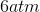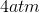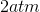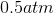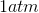Explanation:

Ideal gas law (modified)

P1V1 = P2V2

P1 = 2 atm; V1 = 60L; P2 = ?; V2 = 30L

(2)(60) = (X)(30)

P2 = 4 atm

### Example Question #1 : Gas Laws

Ammonia is created according to the balanced equation below.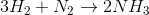The reaction is allowed to take place in a rigid container. Eight moles of hydrogen gas are mixed with two moles of nitrogen gas. The initial pressure exerted on the container is 5atm.

Assuming the reaction runs to completion, what will the pressure exerted on the vessel be after the reaction takes place?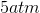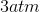Explanation:

Since the total pressure is dependent on the number of moles in the container, we can use the ratio of moles before and after the reaction to determine the final pressure in the container. There are initially ten moles of gas in the container, eight moles of hydrogen and two moles of nitrogen.

The next step is to determine how many moles of ammonia are created in the reaction, and if there is any excess reactant left over after the reaction. Since there is a 1:3 ratio for hydrogen gas to nitrogen gas, only six of the moles of hydrogen gas will be used in order to react will all two moles of the nitrogen gas.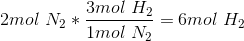This leaves two moles of excess hydrogen gas. Using stoichiometry and the molar ratios, we determine that four moles of ammonia are created in the reaction that comsumes two moles of nitrogen.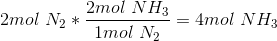Four moles of ammonia, plus the two remaining moles of hydrogen gas, results in six moles of total gas after the reaction has run to completion.

Six moles is 60% of the inital moles in the container, so the final pressure will be 60% of the initial pressure. We can solve using the ideal gas law.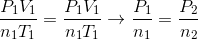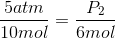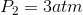### Example Question #11 : Gases

How many moles of Oxygen gas are present at a volume of 10 L at 1 atm and 25o C? (MW Oxygen gas = 32 g/mol)

2.5 mol

0.41 mol

14 mol

4 mol

0.041 mol

0.41 mol

Explanation:

use PV = nRT

n = PV/ RT

P = 1 atm; V = 10 L; R = 0.0821 Latm/molK; T = 298 K (MUST switch temperature to K)

n = moles of gas

n = (1atm)(10L)/(0.0821Latm/molK)(298K)

n= 0.41 mol

### Example Question #1 : Gas Laws

How many moles will a gas that is behaving ideally be if it takes up 2L at 4atm at 37o C?

3.18 mol

0.6 mol

2.63 mol

0.379 mol

0.314 mol

0.314 mol

Explanation:

Use PV = nRT

n = PV/RT

= (2L)(4atm) / (0.0821 Latm/molK)(310 K) <-- must change T into K

= 8/25.451

= 0.314 mol

### Example Question #16 : Gases

If the pressure of a sample of one mole of an ideal gas is increased from 2atm to 3atm at a constant volume, and the initial temperature was 20˚C, what is the final temperature of the sample?

195.3K

303K

439.5K

30K

439.5K

Explanation:

Because the mass and volume of the sample of the ideal gas are kept constant, a change in pressure causes only a direct change in the temperature. This can be derived from the following ideal gas equation:.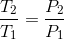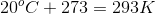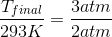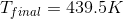### Example Question #2 : Gas Laws

How many moles of carbon dioxide occupy a space of 16 liters at a pressure of 760torr and a temperature of 250K?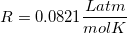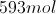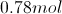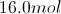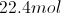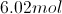Explanation:

We can calculate the number of moles using the ideal gas law: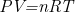Units always have to be in SI units: atm, liters, kelvin, etc. For this question, we have SI units for everything except for the pressure, which is given in torr, rather than atm.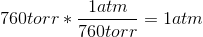Now that we have the proper units, we can use our given values for pressure, temperature, and volume to find the moles of gas present.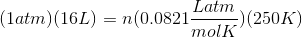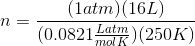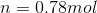### Example Question #18 : Gases

A 2mol sample of an ideal gas with a volume of 3L and temperature of 298K experiences a decrease in pressure of 4atm. If the mass and temperature remain constant, what is the final volume?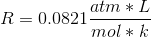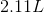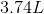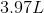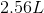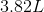Explanation:

First, one must find the initial pressure by plugging the initial conditions into the ideal gas equation.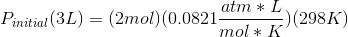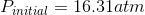Decreasing this by 4 atm leads to a final pressure of 12.31atm, which one can plug into the ideal gas equation to find the final volume.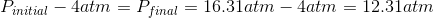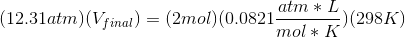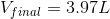Because only the pressure and volume change, one can also use Boyle's law after finding the initial and final pressure values.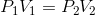### Example Question #1 : Using Gay Lussac's Law

A sample of chlorine gas fills a vessel at a temperature of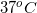. The vessel has a volume of 3L and experiences a pressure of 3atm. What is the mass of the chlorine gas in the vessel?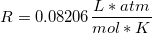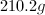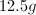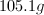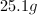Explanation:

This problem requires us to substitute the moles of gas in the ideal gas law to mass over molar mass.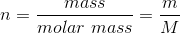We can then isolate for the mass of chlorine gas in the vessel.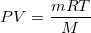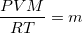Chlorine is diatomic, so its molecular weight will be twice the atomic mass.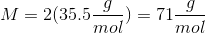The temperature must be expressed in Kelvin.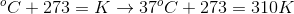Using these values, we can solve for the mass of chlorine gas in the vessel.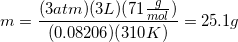← Previous 1

### All AP Chemistry Resources